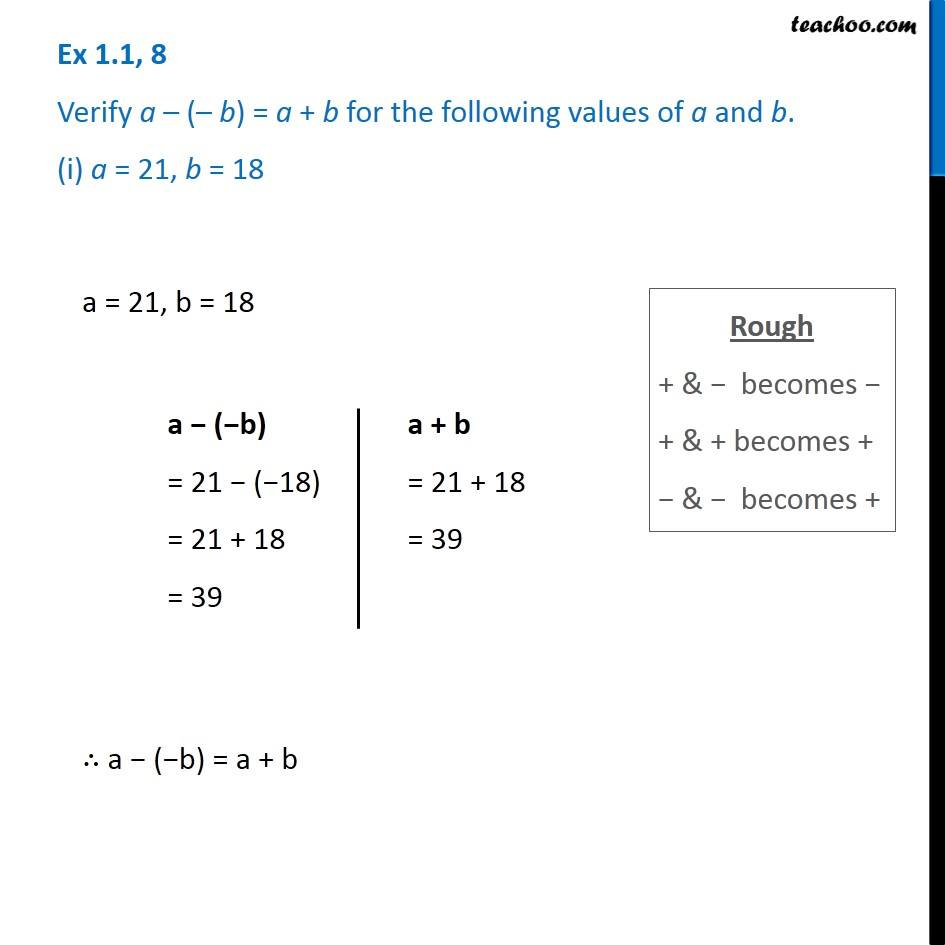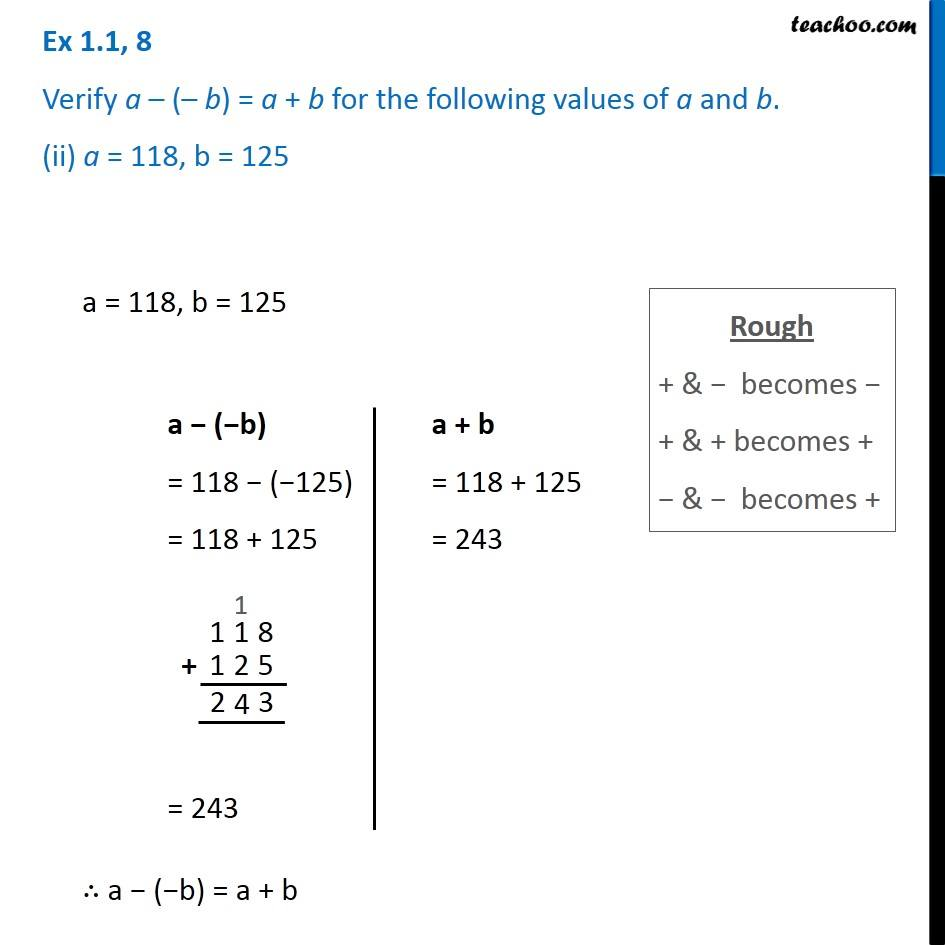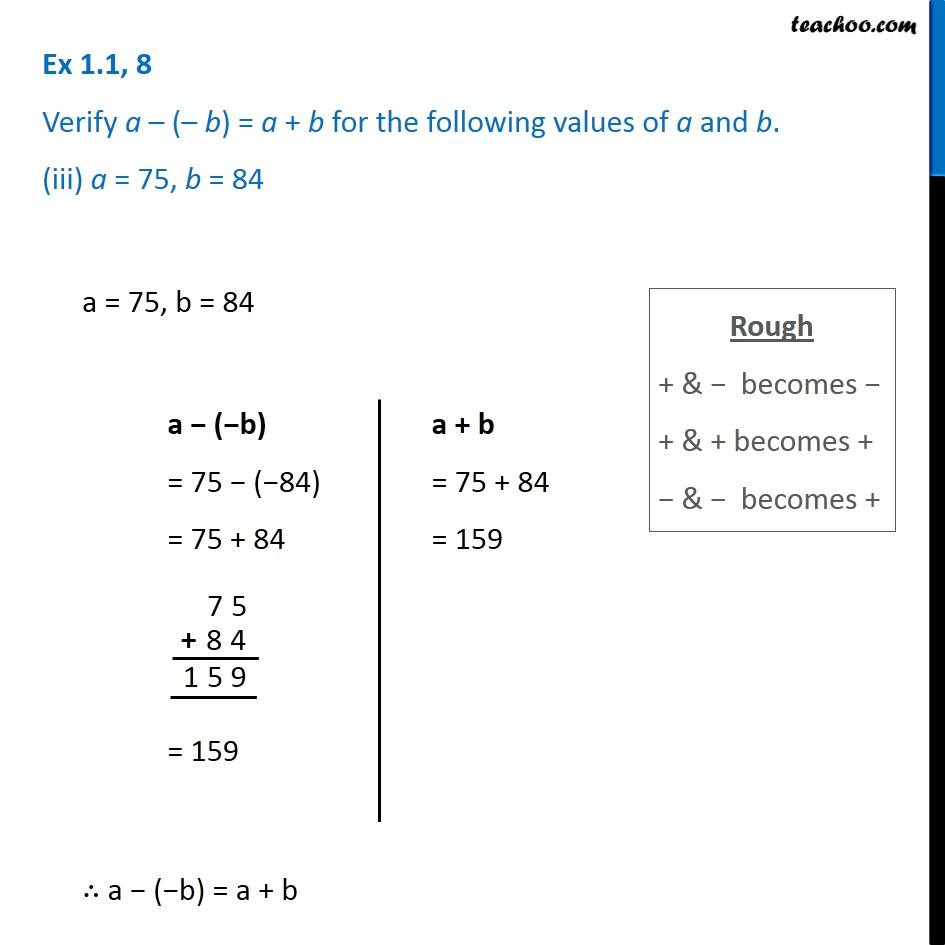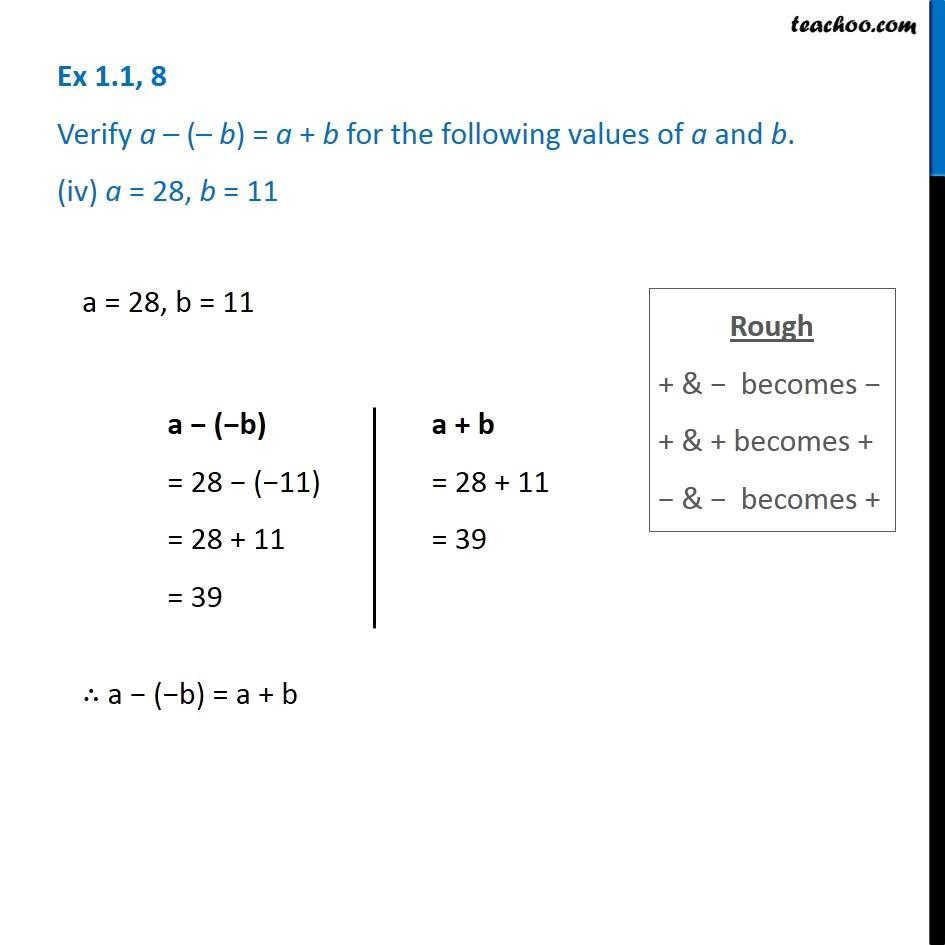Subscribe to our Youtube Channel - https://you.tube/teachoo

1. Chapter 1 Class 7 Integers
2. Serial order wise
3. Ex 1.1

Transcript

Ex 1.1, 8 Verify a – (– b) = a + b for the following values of a and b. (i) a = 21, b = 18 Rough + & − becomes − + & + becomes + − & − becomes + a = 21, b = 18 a − (−b) = 21 − (−18) = 21 + 18 = 39 a + b = 21 + 18 = 39 ∴ a − (−b) = a + b Ex 1.1, 8 Verify a – (– b) = a + b for the following values of a and b. (ii) a = 118, b = 125 Rough + & − becomes − + & + becomes + − & − becomes + a = 118, b = 125 a − (−b) = 118 − (−125) = 118 + 125 = 243 a + b = 118 + 125 = 243 ∴ a − (−b) = a + b Ex 1.1, 8 Verify a – (– b) = a + b for the following values of a and b. (iii) a = 75, b = 84 Rough + & − becomes − + & + becomes + − & − becomes + a = 75, b = 84 a − (−b) = 75 − (−84) = 75 + 84 = 159 a + b = 75 + 84 = 159 ∴ a − (−b) = a + b Ex 1.1, 8 Verify a – (– b) = a + b for the following values of a and b. (iv) a = 28, b = 11 a = 28, b = 11 a − (−b) = 28 − (−11) = 28 + 11 = 39 a + b = 28 + 11 = 39 ∴ a − (−b) = a + b

Ex 1.1

Chapter 1 Class 7 Integers
Serial order wise